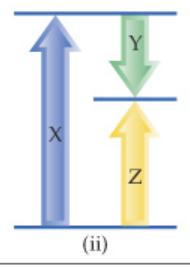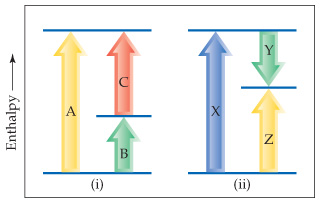# Problem: Consider the two diagrams below.Based on (ii), write an equation relating HZ to the other enthalpy changes in the diagram.

###### FREE Expert Solution

Based on the diagram:$\overline{){\mathbf{∆}}{{\mathbf{H}}}_{{\mathbf{X}}}{\mathbf{=}}{\mathbf{∆}}{{\mathbf{H}}}_{{\mathbf{Z}}}{\mathbf{+}}{\mathbf{∆}}{{\mathbf{H}}}_{{\mathbf{Y}}}}$

88% (347 ratings)###### Problem Details

Consider the two diagrams below.Based on (ii), write an equation relating HZ to the other enthalpy changes in the diagram.